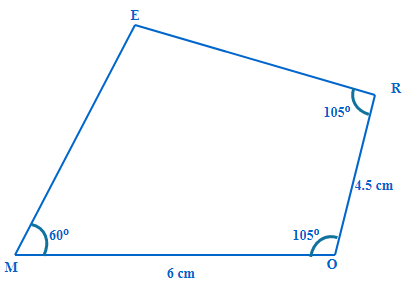# Ex.4.3 Q1 Practical Geometry Solution - NCERT Maths Class 8

Go back to  'Ex.4.3'

## Question

(i) Quadrilateral $$MORE$$

$$MO = 6\,\rm{cm}$$

$$OR = 4.5\,\rm{cm}$$

$$\angle {M = 60 }^\circ$$

$$\angle {O = 105 }^\circ$$

$$\angle R = 105^\circ$$

(ii) Quadrilateral $$PLAN$$

$$PL=4\,\rm{cm}$$

$$LA=6.5\,\rm{cm}$$

$$\angle P=90^\circ$$

$$\angle A=110^\circ$$

$$\angle N=85^\circ$$

(iii) Parallelogram $$HEAR$$

$$HE = 5\,\rm{cm}$$

$$EA = 6\,\rm{cm}$$

$$\angle R = 85^\circ$$

(iv) Rectangle $$OKAY$$

$$OK=7\,\rm{cm}$$

$$KA=5\,\rm{cm}$$

## Text Solution

What is known?

Measurements of two sides and three angles

What is unknown?

Reasoning:

As you are aware, we need five measurements to draw a quadrilateral .

The measurements of two adjacent sides and three angles

Steps:

Let us draw a rough diagram of the quadrilateralStep 1: Draw a line segment $$MO=6 \,\rm{cm}.$$

Step 2: With $$M$$ as center draw angle of measure $$60^\circ.$$

Step 3: Draw $$105^\circ$$ from $$O.$$

Step 4: With $$O$$ as center and radius $$4.5\,\rm{cm}$$ draw an arc cutting the ray from $$O$$ at $$R.$$ $$OR = 4.5\,\rm{cm}$$

Step 5: Construct an angle of $$105^{\circ}$$ from $$R$$ as above. The ray from $$R$$ meets the ray from $$M$$ at a point. Mark the intersection point as $$E.$$

Step 6: $$MORE$$  is the required quadrilateral.

Learn from the best math teachers and top your exams

• Live one on one classroom and doubt clearing
• Practice worksheets in and after class for conceptual clarity
• Personalized curriculum to keep up with school